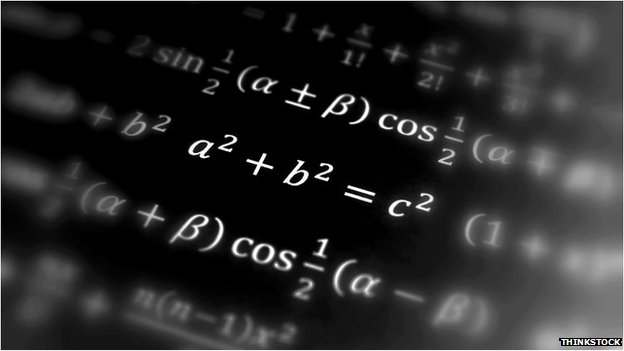# The beauty of mathsBeautiful equations

I reblogged (is that a word?) earlier on today about the importance of mathematical research. Mathematical models can be practical. But many mathematicians will agree that aesthetics is an equal aspect to any mathematical model. That was the 2nd pont of Mathbabe’s post. Brain scans now prove that mathematicians are not making this up.

Advertisements